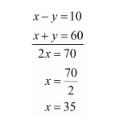# Places A and B are 80 km apart from each other on a highway.Question:

Places A and B are 80 km apart from each other on a highway. A car starts from A and other from B at the same time. If they move in the same direction, they meet in 8 hours and if they move in opposite directions, they meet in 1 hour and 20 minutes. Find the speeds of the cars.

Solution:

Let x and y be two cars starting from points A and B respectively.

Let the speed of the car X be x km/hr and that of the car Y be y km/hr.

Case I: When two cars move in the same directions:

Suppose two cars meet at point Q, then,

Distance travelled by car X = AQ

Distance travelled by car Y = BQ

It is given that two cars meet in 8 hours.

Distance travelled by car $X$ in 8 hours $=8 x \mathrm{~km}$

$A Q=8 x$

Distance travelled by car $Y$ in 8 hours $=8 y \mathrm{~km}$

$\mathrm{BQ}=8 y$

Clearly $A Q-B Q=A B$

$8 x-8 y=80$

Both sides divided by 8, we get

$x-y=10 \cdots(i)$

Case II: When two cars move in opposite direction

Suppose two cars meet at point P, then,

Distance travelled by X car X=AP

Distance travelled by Y car Y=BP

In this case, two cars meet in 1 hour 20 minutes, we can write it as 1 hour $\frac{20}{60}$ or

$1 \frac{1}{3}$ hours that is $\frac{4}{3}$ hours.

Therefore,

Distance travelled by car $y$ in $\frac{4}{3}$ hours $=\frac{4}{3} x \mathrm{~km}$

Distance travelled by car $y$ in $\frac{4}{3}$ hours $=\frac{4}{3} y \mathrm{~km}$

$A P+B P=A B$

$\frac{4}{3} x+\frac{4}{3} y=80$

$\frac{4}{3}(x+y)=80$

$(x+y)=80 \times \frac{3}{4}$

$x+y=60$....(ii)

By solving (i) and (ii) we get,By substituting $x=35$ in equation (ii), we get

$x+y=60$

$35+y=60$

$y=60-35$

$y=25$

Hence, speed of car $X$ is $35 \mathrm{~km} / \mathrm{hr}$, speed of car $Y$ is $25 \mathrm{~km} / / \mathrm{hr}$.